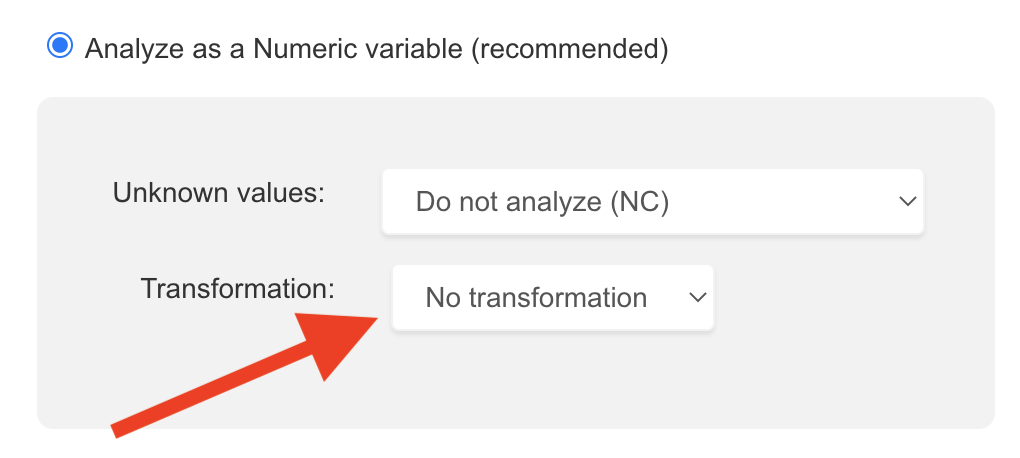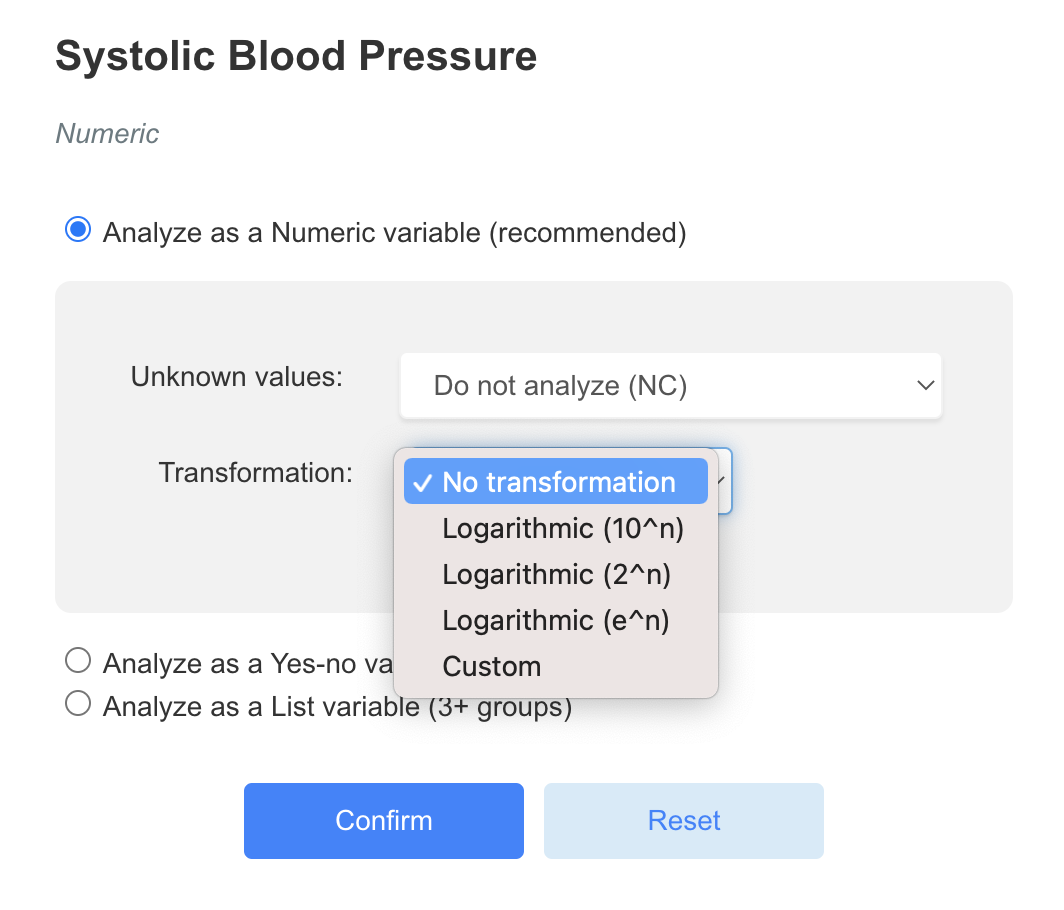# Use Mathematical Expressions to Transform your Variables

Modified on Fri, 19 Aug 2022 at 01:54 PM

When performing statistical tests, you can transform your variables by using mathematical expressions.

One common example is to use the logarithm of a variable. Let's say you are studying a biological parameter with a very large spread from 10 to 1.000.000. Instead of using the raw value, you might want to analyze the logarithm of this value to reduce the range to 1 to 6 instead of 10 to 1.000.000.

## How to use transformations?

1. Click on the cog wheel next to the name of the variable:## Use a logarithmic transformation

Logarithmic transformations are available from the transformation menu as described above.

Just pick the logarithmic transformation you want. Then validate by clicking on the "Confirm" button.Beware! If you have 0 or negative values, the logarithmic transformations will not be applied and an error message will be raised.

## Other mathematical expressions

A wide range of mathematical expressions is available to transform your variables.

To use a custom mathematical expression:

1. Choose "Custom" in the transformation menu
2. Type a formula using "x" for the variable, for example: "x + 100" to add 100 to your variable

### Operators

ExpressionDefinitionComment / Example
+Plusx + 100
-Minus100 - x
*Multiply
/DivideIf the variable data contains 0, an error will be raised and the formula will not be applied
** or ^ or powPowerx ** 2 is x squared
%Modulo
==Is equal to
!=Is not equal to
>Is greater than
<Is lower than
>=Is greater or equal
<=Is lower or equal
andAndTo be used with the "if" expression
if(a, b, c)If "a" then "b" else "c"if(x>=100 and x<=200, x, 100)
If the value of the variable is between 100 and 200, then we use the variable. Else, we define the value as 100.
in(a, b, ...)Belongs toif(x in (1, 2, 5), 0, 1)
If the value of the variable is 1, 2 or 5, then we define the value as 0, else we defined the value as 1
notNotif(not(x in (1, 2, 5), 0, 1)
This expression will have the opposite behaviour of the previous example
orOrif(x==1 or x==2, 0, 1)
If x is 1 or 2, then we define the value as 0, else we define the value as 1
xorExclusive Orhttps://en.wikipedia.org/wiki/Exclusive_or

### Functions

ExpressionDefinitionComment / Example
abs(x)Absolute value
acosd(x)Arc cosinus, x in degrees
asind(x)Arc sinus, x in degrees
atand(x)Arc tangent, x in degrees
atan2(x)Two-arguments arc tangent
ceil(x)Upper-value roundingRounds to the closest integer greater than x
ceil(2.3) = 3
cosd(x)Cosinus, x in degrees
exp(x)Exponential
exp(x, base)Exponential in a specific base
fac(x)Factorial of x
floor(x)Lower-value roundingRounds to the closest integer lower than x
floor(2.3) = 2
log(x)Logarithm
log(x, base)Logarithm in a specific base
max(x1, x2, ..., xn)Maximum value between x1, x2, ...max(x, 100) will always return a value greater or equal to 100
min(x1, x2, ..., xn)Minimum value between x1, x2, ...min(x, 100) will always return a value lower or equal to 100
pyt(x, y)Pythagorian value of x,y:
sqrt(x^2 + y^2)

random(x)Random number in [0, x]
round(x)Integer roundingRounds to the closest integer
round(2.3) = 2
round(2.7) = 3
round(x, n)Rounding to n digits